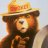# How to scan StochasticSlow converging?

N

#### nyphalanx

##### New member
I'm working on a custom study for a scan and one of the criteria I'm trying to look for is when the slow stochastic K & D line are converging, but I don't know how to write the code. The code I have below tells me when they've crossed, but that's a late signal for me since I want to know when they're starting to converge.

Code:
``````#####
def ignore_stochastic = 0;
def ignore_regression = 0;

# number of trading days to use
input num_of_days = 60;
# % of standard deviation line, where 100 = 1 standard deviation
input regression_line_pct = 90.0;

#####
# average volume for last 50 days is over 1,000,000
def cond_avg_volume = Average("data" = volume, "length" = num_of_days) is greater than 1000000;

#####
# stochastic K moved above stochastic D
def cond_long_stochastic = ignore_stochastic or (StochasticSlow()."SlowK" crosses above StochasticSlow()."SlowD");

#####
# stochastic K moved below stochastic D
def cond_short_stochastic = ignore_stochastic or (StochasticSlow()."SlowK" crosses below StochasticSlow()."SlowD");

#####
# Linear Regression close to 1 std deviation
# Price is above .90% of 1 std deviation lines (top or bottom)
def price_regression = close;
def MiddleLR = InertiaAll(price_regression, num_of_days);
def dist = HighestAll(AbsValue(MiddleLR - price_regression)) * (regression_line_pct / 100.0);
def UpperLR = MiddleLR + dist;
def LowerLR = MiddleLR - dist;

def cond_regression_short = ignore_regression or (price_regression > UpperLR);
def cond_regression_long = ignore_regression or (price_regression < LowerLR);

#####
# Final conditions for long & short
def cond_short = cond_short_stochastic and cond_regression_short;
def cond_long = cond_long_stochastic and cond_regression_long;

plot ScanShort = cond_avg_volume and (cond_short or cond_long);``````Volume scan for past 10 bars on daily and those bars meet another criteria Questions 4